NEET  >  31 Year NEET Previous Year Questions: Motion in a Straight Line - 1

# 31 Year NEET Previous Year Questions: Motion in a Straight Line - 1

Test Description

## 16 Questions MCQ Test Physics Class 11 | 31 Year NEET Previous Year Questions: Motion in a Straight Line - 1

31 Year NEET Previous Year Questions: Motion in a Straight Line - 1 for NEET 2023 is part of Physics Class 11 preparation. The 31 Year NEET Previous Year Questions: Motion in a Straight Line - 1 questions and answers have been prepared according to the NEET exam syllabus.The 31 Year NEET Previous Year Questions: Motion in a Straight Line - 1 MCQs are made for NEET 2023 Exam. Find important definitions, questions, notes, meanings, examples, exercises, MCQs and online tests for 31 Year NEET Previous Year Questions: Motion in a Straight Line - 1 below.
Solutions of 31 Year NEET Previous Year Questions: Motion in a Straight Line - 1 questions in English are available as part of our Physics Class 11 for NEET & 31 Year NEET Previous Year Questions: Motion in a Straight Line - 1 solutions in Hindi for Physics Class 11 course. Download more important topics, notes, lectures and mock test series for NEET Exam by signing up for free. Attempt 31 Year NEET Previous Year Questions: Motion in a Straight Line - 1 | 16 questions in 30 minutes | Mock test for NEET preparation | Free important questions MCQ to study Physics Class 11 for NEET Exam | Download free PDF with solutions
 1 Crore+ students have signed up on EduRev. Have you?
31 Year NEET Previous Year Questions: Motion in a Straight Line - 1 - Question 1

### A particle moving along x-axis has acceleration f, at time t, given by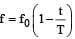, where f0 and T are constants. The particle at t = 0 has zero velocity. In the time interval between t = 0 and the instant when f = 0, the particle’s velocity (vx) is 

Detailed Solution for 31 Year NEET Previous Year Questions: Motion in a Straight Line - 1 - Question 1

Here,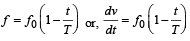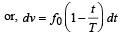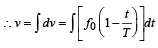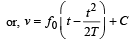where C is the constant of integration.
At t = 0, v = 0.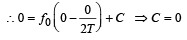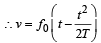If f = 0, then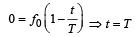Hence, particle's velocity in the time interval t = 0 and t = T is given by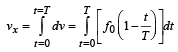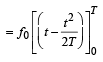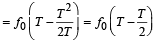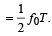31 Year NEET Previous Year Questions: Motion in a Straight Line - 1 - Question 2

### The distance travelled by a particle starting from rest and moving with an acceleration 4/3ms-2 , in the third second is:

Detailed Solution for 31 Year NEET Previous Year Questions: Motion in a Straight Line - 1 - Question 2

Distance travelled in the nth second is given by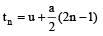put u = 0,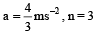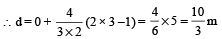31 Year NEET Previous Year Questions: Motion in a Straight Line - 1 - Question 3

###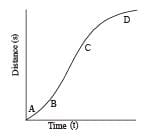A particle shows distance - time curve as given in this figure. The maximum instantaneous velocity of the particle is around the point:                              

Detailed Solution for 31 Year NEET Previous Year Questions: Motion in a Straight Line - 1 - Question 3

The slope of the graph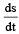is maximum at C and hence the instantaneous velocity is maximum at C.

31 Year NEET Previous Year Questions: Motion in a Straight Line - 1 - Question 4

A particle moves in a straight line with a constant acceleration. It changes its velocity from 10 ms–1 to 20 ms–1 while passing through a distance 135 m in t second. The value of t is: 

Detailed Solution for 31 Year NEET Previous Year Questions: Motion in a Straight Line - 1 - Question 4

Initial velocity, u = 10 ms–1
Final velocity, v = 20 ms–1
Distance, s = 135 m
Let, acceleration = a
Using the formula, v2 = u2 + 2as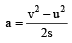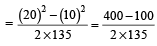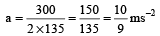Now, using the relation, v = u + at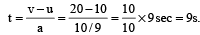31 Year NEET Previous Year Questions: Motion in a Straight Line - 1 - Question 5

A bus is moving with a speed of 10 ms–1 on a straight road. A scooterist wishes to overtake the bus in 100 s. If the bus is at a distance of 1 km from the scooterist, with what speed should the scooterist ch ase the bus? 

Detailed Solution for 31 Year NEET Previous Year Questions: Motion in a Straight Line - 1 - Question 5

Let v be the relative velocity of scooter w.r.t bus as v = vS– vB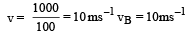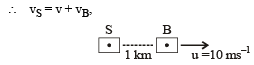=   10 + 10 = 20 ms–1

∴ velocity of scooter = 20 ms–1

31 Year NEET Previous Year Questions: Motion in a Straight Line - 1 - Question 6

A particle starts its motion from rest under the action of a constant force. If the distance covered in first 10 seconds is S1 and that covered in the first 20 seconds is S2, then: 

Detailed Solution for 31 Year NEET Previous Year Questions: Motion in a Straight Line - 1 - Question 6

u = 0, t1=10s, t2 = 20s

Using the relation,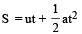Acceleration being the same in two cases,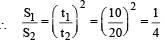S2 = 4S1

31 Year NEET Previous Year Questions: Motion in a Straight Line - 1 - Question 7

A ball is dropped from a high rise platform at t = 0 starting from rest. After 6 seconds another ball is thrown downwards from the same platform with a speed v. The two balls meet at t = 18s.What is the value of v?  (take g = 10 m/s2)

Detailed Solution for 31 Year NEET Previous Year Questions: Motion in a Straight Line - 1 - Question 7

Clearly distance moved by 1st bal l in 18s = distance moved by 2nd ball in 12s.
Now, distance moved in 18 s by 1st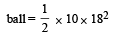= 90 x 18 = 1620 m

Distance moved in 12s by 2nd ball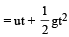∴ 1620 = 12 v + 5 x 144

⇒    v = 135 – 60 = 75 ms –1

31 Year NEET Previous Year Questions: Motion in a Straight Line - 1 - Question 8

A particle h as in itial velocity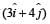and has acceleration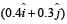. It's speed after 10 s is:

Detailed Solution for 31 Year NEET Previous Year Questions: Motion in a Straight Line - 1 - Question 8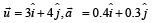⇒ ux = 3 units, uy = 4 units

ax = 0.4 units, ay = 0.3 units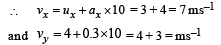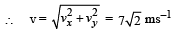31 Year NEET Previous Year Questions: Motion in a Straight Line - 1 - Question 9

A particle moves a distance x in time t according to equation x = (t + 5)-1. The acceleration of particle is proportional to 

Detailed Solution for 31 Year NEET Previous Year Questions: Motion in a Straight Line - 1 - Question 9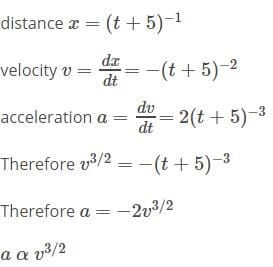31 Year NEET Previous Year Questions: Motion in a Straight Line - 1 - Question 10

A man of 50 kg mass is standing in a gravity free space at a height of 10 m  above the floor. He throws a stone of 0.5 kg  mass downwards with a speed 2 m/s. When the stone reaches the floor, the distance of the man above the floor will be:

Detailed Solution for 31 Year NEET Previous Year Questions: Motion in a Straight Line - 1 - Question 10

No external force is acting, there fore, momentum is conserved.
By momentum conservation, 50u + 0.5 × 2 = 0 where u is the velocity of man.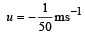Negative sign of u shows that man moves upward.
Time taken by the stone to reach the ground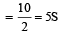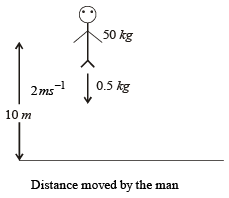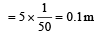∴ when the stone reaches the floor, the distance of the man above floor = 10.1 m

31 Year NEET Previous Year Questions: Motion in a Straight Line - 1 - Question 11

A boy standing at the top of a tower of 20m height drops a stone. Assuming g = 10 ms–2, the velocity with which it hits the ground is 

Detailed Solution for 31 Year NEET Previous Year Questions: Motion in a Straight Line - 1 - Question 11

Here, u = 0

We have, v2 = u2 + 2gh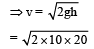= 20 m/s

31 Year NEET Previous Year Questions: Motion in a Straight Line - 1 - Question 12

A body is moving with velocity 30 m/s towards east. After 10 seconds its velocity becomes 40 m/s towards north. The average acceleration of the body is 

Detailed Solution for 31 Year NEET Previous Year Questions: Motion in a Straight Line - 1 - Question 12

Average acceleration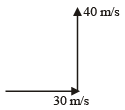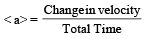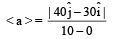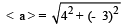< a > = 5 m/sec2

31 Year NEET Previous Year Questions: Motion in a Straight Line - 1 - Question 13

A particle covers half of its total distance with speed v1 and the rest half distance with speed v2. Its average speed during the complete journey is

Detailed Solution for 31 Year NEET Previous Year Questions: Motion in a Straight Line - 1 - Question 13

Let the total distance covered by the particle be 2s. Then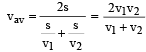31 Year NEET Previous Year Questions: Motion in a Straight Line - 1 - Question 14

The motion of a particle along a straight line is described by equation :  x = 8 + 12t – t3 where x is in metre and t in second. The retardation of the particle when its velocity becomes zero, is :

Detailed Solution for 31 Year NEET Previous Year Questions: Motion in a Straight Line - 1 - Question 14

x = 8 + 12t – t3
The final velocity of the particle will be zero, because it retarded.
V = 0 + 12 – 3t2 = 0
3t2 = 12
t = 2 sec
Now the retardation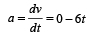a [t = 2] = – 12 m/s2 retardation = 12 m/s2

31 Year NEET Previous Year Questions: Motion in a Straight Line - 1 - Question 15

A stone falls freely  under gravity. It covers distances h1, h2 and h3 in the first 5 seconds, the next 5 seconds and the next 5 seconds respectively. The relation between h1, h2 and h3 is [NEET 2013]

Detailed Solution for 31 Year NEET Previous Year Questions: Motion in a Straight Line - 1 - Question 15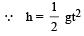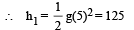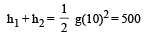⇒  h2 = 375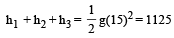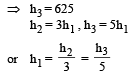31 Year NEET Previous Year Questions: Motion in a Straight Line - 1 - Question 16

The displacement ‘x’ (in meter) of a particle of mass ‘m’ (in kg) moving in one dimension under the action of a force, is related to time ‘t’ (in sec) by t =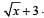. The displacement of the particle when its velocity is zero, will be [NEET Kar. 2013]

Detailed Solution for 31 Year NEET Previous Year Questions: Motion in a Straight Line - 1 - Question 16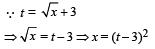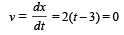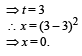## Physics Class 11

127 videos|464 docs|210 tests
 Use Code STAYHOME200 and get INR 200 additional OFF Use Coupon Code
Information about 31 Year NEET Previous Year Questions: Motion in a Straight Line - 1 Page
In this test you can find the Exam questions for 31 Year NEET Previous Year Questions: Motion in a Straight Line - 1 solved & explained in the simplest way possible. Besides giving Questions and answers for 31 Year NEET Previous Year Questions: Motion in a Straight Line - 1 , EduRev gives you an ample number of Online tests for practice

## Physics Class 11

127 videos|464 docs|210 tests

### How to Prepare for NEET

Read our guide to prepare for NEET which is created by Toppers & the best Teachers